Math Calculators, Lessons and Formulas

It is time to solve your math problem

mathportal.org
Linear Algebra - Vectors: (lesson 2 of 3)

## Dot Product

Definition:

The dot product (also called the inner product or scalar product) of two vectors is defined as: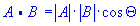Where |A| and |B| represents the magnitudes of vectors A and B and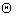is the angle between vectors A and B.

### Dot product calculation

The dot or scalar product of vectors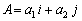and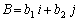can be written as: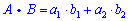Example (calculation in two dimensions):

Vectors A and B are given by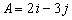and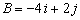. Find the dot product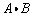of the two vectors.

Solution: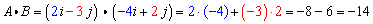Example (calculation in three dimensions):

Vectors A and B are given by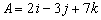and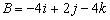. Find the dot productof the two vectors.

Solution: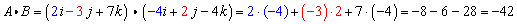### Calculating the Length of a Vector

The length of a vectoris: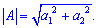Example:

Vector A is given by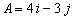. Find |A|.

Solution: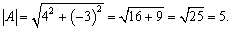### The angle between two vectors

The angle between two nonzero vectors A and B is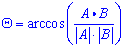Example: (angle between vectors in two dimensions):

Determine the angle between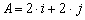and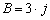.

Solution:

We will need the magnitudes of each vector as well as the dot product.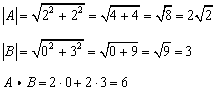The angle is,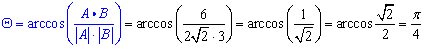Example: (angle between vectors in three dimensions):

Determine the angle between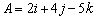and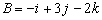.

Solution:

Again, we need the magnitudes as well as the dot product.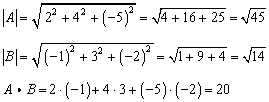The angle is,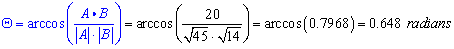### Orthogonal vectors

If two vectors are orthogonal then: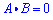.

Example:

Determine if the following vectors are orthogonal: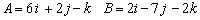Solution:

The dot product is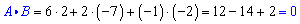So, the two vectors are orthogonal.